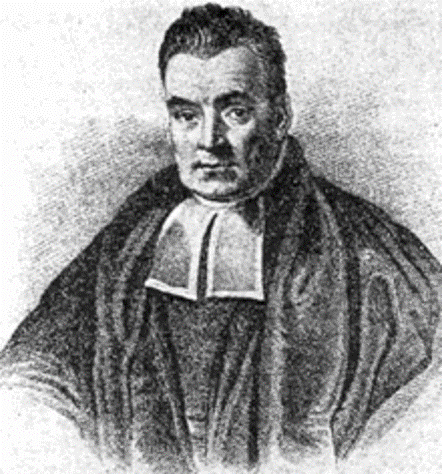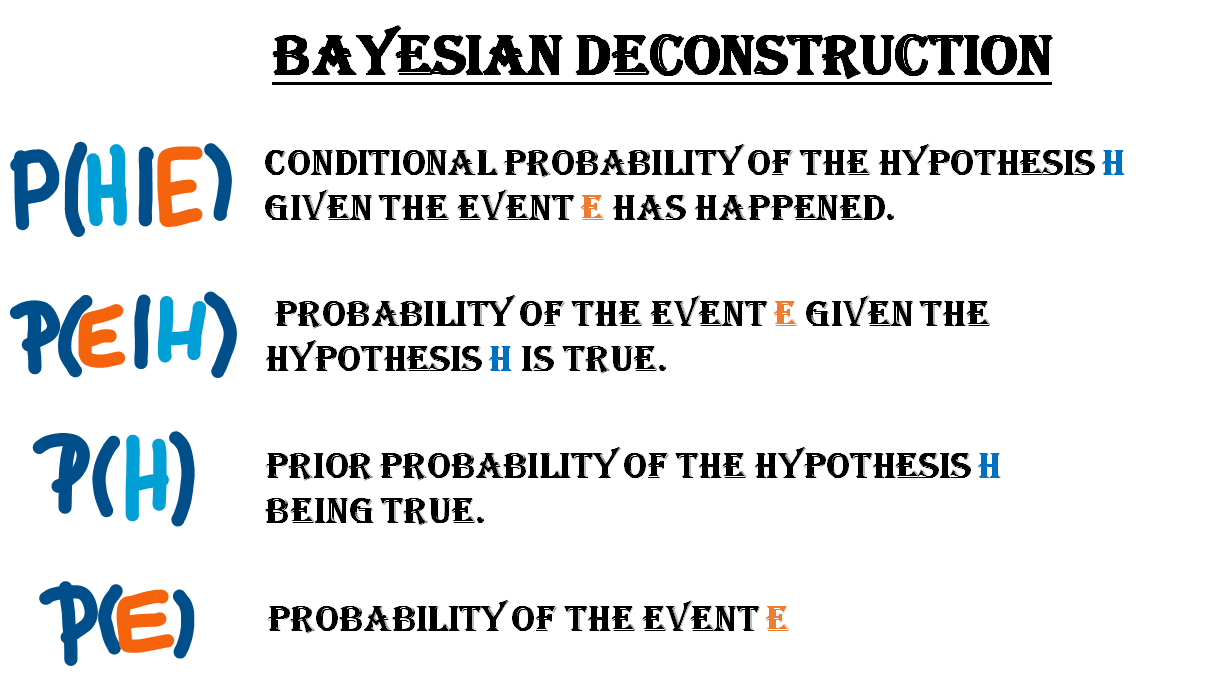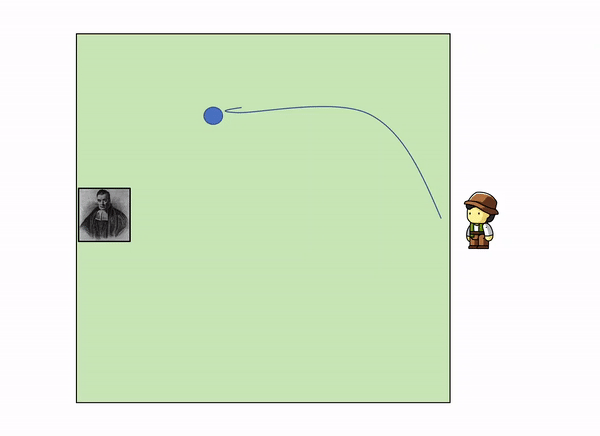Topics: AI | Data Science | Data Visualization | Deep Learning | Machine Learning | NLP | Python | R | Statistics

KDnuggets Home » News » 2019 » Oct » Tutorials, Overviews » Probability Learning: Bayes’ Theorem ( 19:n40 )

# Probability Learning: Bayes’ Theorem

Learn about one of the fundamental theorems of probability with an easy everyday example.This post assumes you have some basic knowledge of probability and statistics. If you don’t, do not be afraid, I have gathered a list of the best resources I could find to introduce you to these subjects, so that you can read this post, understand it, and enjoy it to its fullest.

In it, we will talk about one of the most famous and utilised theorems of probability theory: Bayes’ Theorem. Never heard about it? Then you are in for a treat! Know what it is already? Then read on to consolidate your knowledge with an easy, everyday example, so that you too can explain it in simple terms to others.

In the following posts we will learn about some simplifications of Baye’s theorem that are more practical, and about other probabilistic approaches to machine learning like Hidden Markov Models.
Lets go!

### Introduction to probability:

In this section I have listed three very good and concise (the two first mainly, the third one is a bit more extensive) sources to learn the basics of probability that you will need to understand this post. Don’t be afraid, the concepts are very simple and with a quick read you will most definitely understand them.

If you already have a grasp of basic probability, feel free to skip this section.

Okay, now you are ready to continue on with the rest of the post, sit back, relax, and enjoy.

### Who was Bayes?

Thomas Bayes (1701 — 1761) was an English theologian and mathematician that belonged to the Royal Society (the oldest national scientific society in the world and the leading national organisation for the promotion of scientific research in Britain), where other eminent individuals have enrolled, like Newton, Darwin or Faraday. He developed one of the most important theorems of probability, which coined his name: Bayes’ Theorem, or the Theorem of Conditional Probability.Portrait of the Reverend Thomas Bayes, father of Bayes’ Theorem

### The Theorem: Conditional Probability

To explain this theorem, we will use a very simple example. Imagine you have been diagnosed with a very rare disease, which only affects 0.1% of the population; that is, 1 in every 1000 persons.

The test you have taken to check for the disease correctly classifies 99% of the people who have the disease, incorrectly classifying healthy individuals with a 1% chance.

I am doomed! Is this disease fatal doctor?

Is what most people would say. However, after this test, what are the chances we have of actually suffering the disease?

99% for sure! I better get my stuff in order.

Upon this thought, Bayes’ mentality ought to prevail, as it is actually very far off from reality. Lets use Bayes’ Theorem to gain some perspective.

Bayes’ Theorem, or as I have called it before, the Theorem of Conditional Probability, is used for calculating the probability of a hypothesis (H) being true (ie. having the disease) given that a certain event (E) has happened (being diagnosed positive of this disease in the test). This calculation is described using the following formulation:Bayes’ formula for conditional probability

The term on the left of the equal sign P(H |E )is the probability of having the disease (H) given that we have been diagnosed positive (E) in a test for such disease, which is what we actually want to calculate. The vertical bars (|) in a probability term denote a conditional probability (ie, the probability of A given B would be P(A|B)).

The left term of the numerator on the right side P(E|H) is the probability of the event, given that the hypothesis is true. In our example this would be the probability of being diagnosed positive in the test, given that we have the disease.

The term next to it; P(H) is the prior probability of the hypothesis before any event has taken place. In this case it would be the probability of having the disease before any test has been taken.

Lastly, the term on the denominator; P(E) is the probability of the event, that is, the probability of being diagnosed positive for the disease. This term can be further decomposed into the sum of two smaller terms: having the disease and testing positive plus not having the disease and testing positive too.Deconstruction of the probability of testing positive on the test

In this formula P(~H) denotes the prior probability of not having the disease, where ~ means negation or not. The following picture describes each of the terms involved in the overall calculation of the conditional probability:Description of each of the terms involved on the formulation of by Bayes’ Theorem

Remember, for us the hypothesis or assumption H is having the disease, and the event or evidence E is being diagnosed positive in the test for such disease.

If we use the first formula we saw (the complete formula for calculating the conditional probability of having the disease and being diagnosed positive), decompose the denominator, and insert in the numbers, we get the following calculation:Calculation of the conditional probability

The 0,99 comes from the 99% probability of being diagnosed positive given that we have the disease, the 0,001 comes from the 1 in 1000 chance of having the disease, the 0,999 comes from the probability of not having this disease, and the last 0,01 comes from the probability of being diagnosed positive by the test even tough we do not have the disease. The final result of this calculation is:Result of the calculation

9%! There is only a 9% chance that we have the disease! “How can this be?” you are probably asking yourself. Magic? No, my friends, it is not magic, it is just probability: common sense applied to mathematics. Like described in the book Thinking, Fast and Slow by Daniel Kahneman, the human mind is very bad at estimating and calculating probabilities, like it has been shown with the previous example, so we should always hold back our intuition, take a step back and use all the probability tools at our disposal.

Imagine now, that after being diagnosed positive on the first test, we decide to take another test with the same conditions, in a different clinic to double-check the results, and unluckily we get a positive diagnosis again, indicating that the second test also says that we have the disease.

What is the actual probability of having the disease now? Well, we can use exactly the same formula as before, but replacing the initial prior probability (0.1% chance of having the disease) with the posterior probability obtained the previous time (9% chance after being diagnosed positive by the test once), and their complementary terms.

If we crunch the numbers we get:Calculation of the conditional probability after the second positiveResults after the second positive

Now we have a much higher chance, 91% of actually having the disease. As bad as it might look tough, after two positive tests it is still not completely certain that we have the disease. Certainty seems to escape the world of probability.

### The intuition behind the theorem

The intuition behind this famous theorem is that we can never be fully certain of the world, as it is a changing being, change is in the nature of reality. However, something that we can do, which is the fundamental principle behind this theorem, is update and improve our knowledge of reality as we get more and more data or evidence.

This can be illustrated with a very simple example. Imagine the following situation: you are in the edge square shaped garden, sitting on a chair, looking outside the garden. On the opposite side, lies a servant that throws a initial blue ball inside the square. After that, he keeps throwing other yellow balls inside the square, and telling you where they land relatively to the initial blue ball.Video of this mental experiment with our good old Bayes sitting on the edge of his garden with his back towards a servant that is throwing the balls.

As more and more yellow balls land, and you get informed of where they land relative to the first blue ball, you gradually increase your knowledge of where the blue ball might be, leaving out certain parts of the garden: as we get more evidence (more yellow balls) we update our knowledge (the position of the blue ball).

In the example above, with only 3 yellow balls thrown, we could already start to build a certain idea that the blue ball lies somewhere within the top left corner of the garden.

When Bayes’ first formulated this theorem, he didn’t publish it at first, thinking it was nothing extraordinary, and the papers where the theorem was formulated were found after his death.

Today Bayes’ theorem is not only one of the bases of modern probability, but a highly used tool in many intelligent systems like spam filters, and many other text and non text related problem solvers.

In the following post we will see what these applications are, and how Bayes’ theorem and its variations can be applied to many real world use cases. To check it out follow me on Medium, and stay tuned!That is all, I hope you liked the post. Feel Free to connect with me on LinkedIn or follow me on Twitter at @jaimezorno. Also, you can take a look at my other posts on Data Science and Machine Learning here. Have a good read!

Bio: Jaime Zornoza is an Industrial Engineer with a bachelor specialized in Electronics and a Masters degree specialized in Computer Science.

Original. Reposted with permission.

Related:

Top Stories Past 30 Days
Most SharedGet KDnuggets, a leading newsletter on AI, Data Science, and Machine Learning